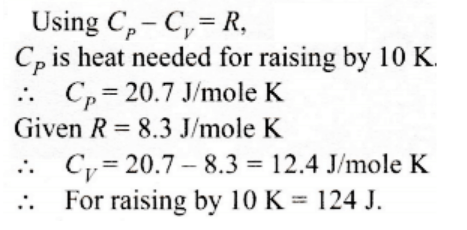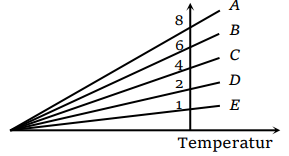## Kinetic Theory Questions and Answers Part-30

1. Inside a cylinder having insulating walls and closed at ends is a movable piston, which divides the cylinder into two compartments. On one side of the piston is a mass m of a gas and on the other side a mass 2 m of the same gas. What fraction of volume of the cylinder will be occupied by the larger mass of the gas when the piston is in equilibrium ? Consider that the movable piston is conducting so that the temperature is the same throughout
a) $\frac{1}{4}$
b) $\frac{1}{3}$
c) $\frac{1}{2}$
d) $\frac{2}{3}$

Explanation:2. The diameter of oxygen molecule is $2.94\times10^{ -10}m$    . The Vander Waal’s gas constant ‘b’ in $m^{ 3}\diagup mol$   will be
a) 3.2
b) 16
c) $32\times10^{ -4}$
d) $32\times10^{ -6}$

Explanation: $32\times10^{ -6}$

3. The temperature of the mixture of one mole of helium and one mole of hydrogen is increased from $0^{\circ} C$  to $100^{\circ} C$  at constant pressure. The amount of heat delivered will be
a) 600 cal
b) 1200 cal
c) 1800 cal
d) 3600 cal

Explanation: 1200 cal

4. A vessel contains a mixture of one mole of oxygen and two moles of nitrogen at 300 K. The ratio of the average rotational kinetic energy per $O_{2}$ molecule to that per $N_{2}$ molecule is
a) 1 : 1
b) 1 : 2
c) 2 : 1
d) Depends on the moments of inertia of the two molecules

Explanation: A vessel contains a mixture of one mole of oxygen and two moles of nitrogen at 300 K. The ratio of the average rotational kinetic energy per $O_{2}$ molecule to that per $N_{2}$ molecule is 1 : 1

5. A vessel contains 14 gm (7 moles) of hydrogen and 96 gm (9 moles) of oxygen at STP. Chemical reaction is induced by passing electric spark in the vessel till one of the gases is consumed. The temperature is brought back to it's starting value 273 K. The pressure in the vessel isa) 0.1 atm
b) 0.2 atm
c) 0.3 atm
d) 0.4 atm

Explanation: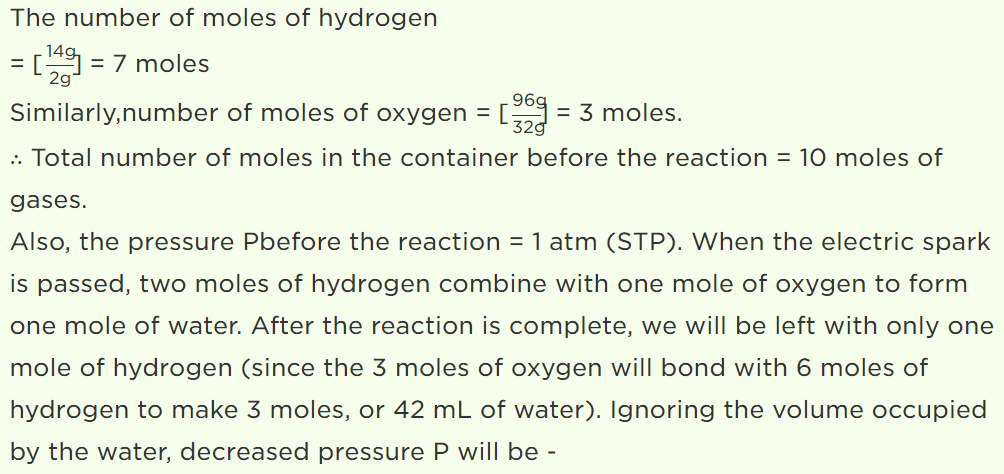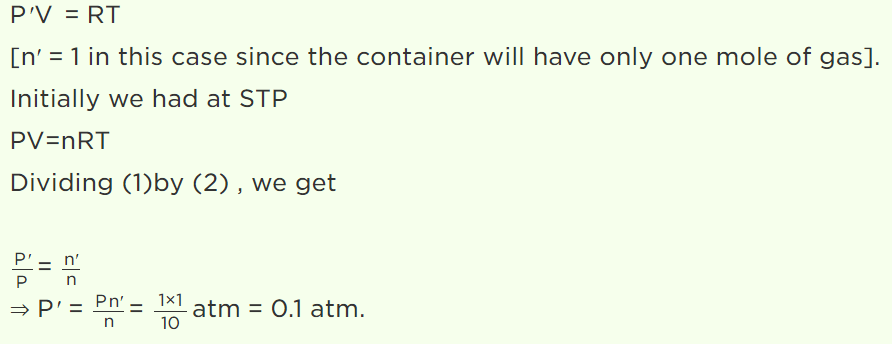6.A gas mixture consists of 2 mole of oxygen and 4 mole of argon at temperature T. Neglecting all vibrational modes, the total internal energy of the system is
a) 4 RT
b) 15 RT
c) 9 RT
d) 11 RT

Explanation: A gas mixture consists of 2 mole of oxygen and 4 mole of argon at temperature T. Neglecting all vibrational modes, the total internal energy of the system is 11 RT

7. A jar contains a gas and few drops of water at T K. The pressure in the jar is 830 mm of mercury. The temperature of jar is reduced by 1%. The saturated vapour pressure of water at the two temperatures are 30 mm and 25 mm of mercury. Then the new pressure in the jar will be
a) 917 mm of Hg
b) 717 mm of Hg
c) 817 mm of Hg
d) None of these

Explanation: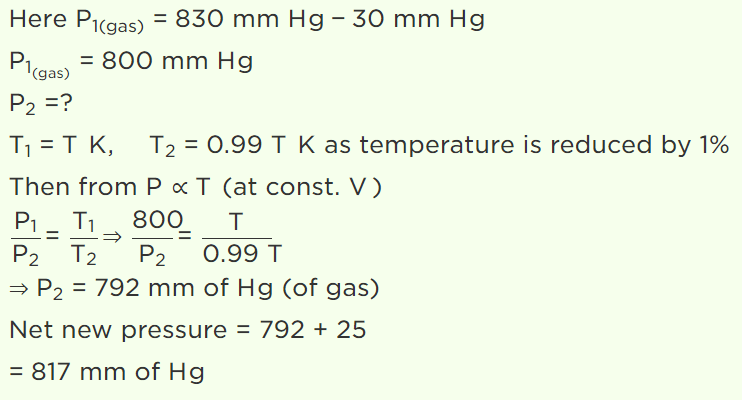8. Molar specific heat of oxygen at constant pressure $C_{P}= 7.2 cal \diagup mol^{\circ} C$     and R = 8.3 joule/mol/K. At constant volume, 5 mol of oxygen is heated from 10°C to 20°C, the quantity of heat required is approximately
a) 25 cal
b) 50 cal
c) 250 cal
d) 500 cal

Explanation: 250 cal

9. One mole of an ideal gas requires 207 J heat to raise the temperature by 10 K when heated at constant pressure. If the same gas is heated at constant volume to raise the temperature by the same 10 K, the heat required is
a) 198.7 J
b) 29 J
c) 215.3 J
d) 124 J